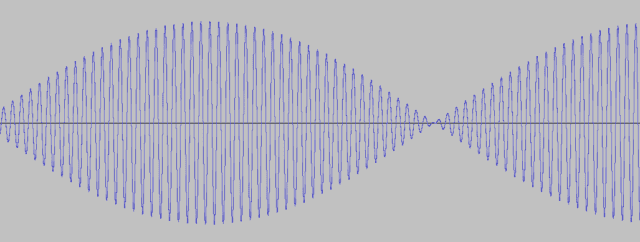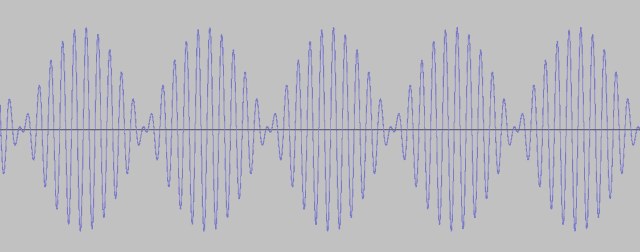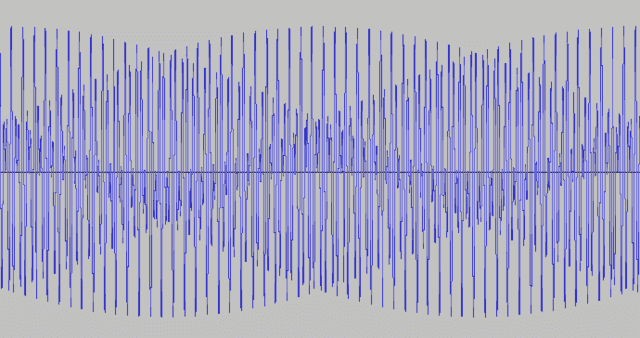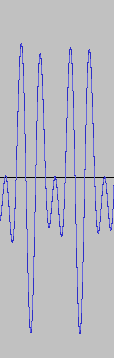# Acoustic ‘beats’ from Mismatched Musical Frequencies

[Total: 1    Average: 5/5]

In late high school physics courses and first year university courses, the phenomenon of acoustical ‘beats’ is often covered. This is an interesting phenomenon, but it is usually only skated over, leaving the students with not much more than a feeling that the beats somehow arise from the two waves moving in and out of sync over time, and thereby generating constructive and destructive interference. That creates the ‘beats’ effect which is, in a very loose sense, the acoustic analogue of the striped interference pattern seen in a double-slit experiment. The attentive student will come away from such instruction remembering that the frequency of the beats is equal to the difference of the two frequencies, but not much more. This short note aims to present a mathematical explanation of the phenomenon, and to observe some of the consequences of the analysis.

Imagine two pure musical tones being played which, observed at a particular point in space give pressure variations (relative to ambient pressure) over time according to the equations:
\begin{equation}P1(t)=\cos (2\pi f_1 t);\ \ P2(t)=\cos (2\pi f_2 t)\end{equation}
The volumes of these waves are the same, the frequencies in Hertz (cycles per second) are ${f_1}$ and ${f_2}$ respectively, and we assume $f_1>f_2$. The ‘periods’, which are the times taken for a full cycle of pressure variation, are $T_1=1/{f_1}$ and $T_2=1/{f_2}$.

At the observation location, the observed pressure variation is the sum of these two pressure functions:
\begin{equation}P_{tot}(t)=\cos (2\pi f_1 t)+\cos (2\pi f_2 t) \end{equation}

We call the argument to the cosine function the ‘phase’ of the wave. The difference between the two phases is called the ‘phase difference’, and it is that which is crucial in understanding beats. We say that the two waves are synchronised at time $t$ (‘in sync’) if the phase difference is a multiple of $2\pi$. That means that the two waves are at the same point in their respective cycles, eg both at a maximum, both at a minimum, both crossing the horizontal axis in an upwards direction, etc. At a time when the two waves are close to ‘in sync’, their amplitudes add, making the pressure variations approximately double the size of those of each wave. That makes the sound louder.

Conversely, when the phase difference is a half-cycle, the pressure variation contributed by one wave will be the negative of that contributed by the other. So the addition will cause them to cancel, and the amplitude of the pressure variations will be close to zero. That makes the sound quieter.

At least, that’s the general idea. Now let’s examine how the mathematics works.

The waves start off in sync at time 0. Then they will be in sync again at all times $t$ such that
\begin{equation}t=a\,{T_2} =(a+k)\,T1 \end{equation}
for integer $k$. The real number $a$ is the number of ${T_2}$ cycles elapsed at time $t$. Solving the equation for $a$ gives $a=k\frac{T1}{{T_2}-T1}$. Setting $k$ equal to 1, we see that the time between successive synchronisations (the ‘synchronisation period’) is:
\begin{equation}T_{sync}\equiv \frac{T1\,{T_2}}{{T_2}-T1}=\frac{1}{1/T1-1/{T_2}}=\frac{1}{{f_1}-{f_2}} \end{equation}
The frequency $f_{sync}$ of synchronisations is the reciprocal of that period, which is ${f_1}-{f_2}$. Around the time of synchronisation, the amplitude of the combined wave is close to double that of the component waves. That high amplitude lasts for as long as the waves are approximately synchronised, and the phenomenon recurs every $T_{sync}$ seconds, generating a surge of volume that we call a ‘beat’, around each synchronisation time.

Consider what would happen if we played two tones with frequencies of 50 Hz and 55 Hz which are, by the way, respectively the second lowest ‘A’ on a standard piano and the hum made by an electrical transformer. We would hear five ‘beats’ per second, as $55-50=5$. If the frequencies were 50 Hz and 51 Hz we would hear only one beat per second. If they were 49 Hz and 54 Hz we would again hear five beats per second.

This is what the wave-form of the 50 Hz vs 51 Hz pair looks like. The height of the blue line above the horizontal line shows the variation over time of pressure above and below the ambient pressure.The following sound clip shows what it sounds like.

And here are the two constituent waves. Unless you are exceptionally talented, you will not be able to tell the difference between the 50Hz and the 51Hz wave. It’s hard to believe that two such pure tones generate the strange sound above.

50Hz:

51Hz:

Considered in terms of levels of pressure variation, there is an interesting difference between the cases of 50 vs 55 and 49 vs 54. For the first case, the first beat will occur at time $t=\frac{1}{5}=\frac{10}{50}=\frac{11}{55}$. That is after 10 and 11 cycles of the lower and higher frequency waves respectively. Further, the synchronisation occurs when the phases are an exact multiple of $2\pi$, so that the pressure variation at that time is exactly the sum of the two amplitudes, maximising the impact.

However, when the waves have frequencies of 49 Hz and 54 Hz, at the time when the first synchronisation occurs at 200 ms, the lower tone has completed 9.8 cycles and the higher tone has completed 10.8. So neither is giving a maximum pressure variation. That happens 4 ms later when the higher frequency wave attains its maximum, having completed 11 cycles. But at that point the waves are slightly out of sync again, so the pressure variation is less than the sum of the amplitudes.

They are not very far out of sync. At 204 ms the lower tone has completed 9.98 cycles, making it one-fiftieth cycle out of sync ($1/50=1/(10-9.98)$), so that the pressure achieved at that time is 1.99 times the individual amplitudes, rather than double. At the next synchronisation after that, the lower tone will be two-fiftieth cycles out of sync at its closest maximum to the synchronisation point. For the next sync after that, the closest maximum for the higher tone is 0.4 cycles before the sync point. The next is 0.2 cycles before and the next sync after that occurs at a joint maximum. This is shown in the following table.
$$\begin{vmatrix} \textit{Sync number }&0 & 1 & 3 & 3 & 4 & 5\\ \textrm{Sync time (ms) }&0 & 200 & 400 & 600 & 800 & 1000\\ \textrm{Wave 1 Phase}&0 & 10.8 & 21.6 & 32.4 & 43.2 & 54.0\\ \textrm{Phase adj to closest-}&0 & 0.2 & 0.4 & -0.4 & -0.2 & 0\\ \textrm{wave 1 max}\\ \textrm{Time of closest- }&0 & 204 & 407 & 593 & 796 & 1000\\ \textrm{wave 1 max (ms)}\\ \textrm{Wave 2 height at that time}&1 & 0.99 & 0.97 & 0.97 & 0.99 & 1\\ \textrm{Total pressure variation}&2 & 1.99 & 1.97 & 1.97 & 1.99 & 2 \end{vmatrix}$$
Looking at the last row of the table, we see that this generates a very small (probably not discernible to the naked ear) cycle of the loudness of the beats, with a period of one second. In general, the discernibility of the variation in loudness will depend on how far away from maximum the pressure wave of the lower tone is when the higher tone is at the closest maximum to a synchronisation time.

To have beats that are constant in volume, we require that, at each synchronisation, both waves are delivering a maximum pressure variation, which means they must have both completed a whole number of cycles. So we require that, at the first synchronisation time $\frac{T1\,T_2}{T_1+T_2}$ we have:
\begin{equation}\frac{T1\,T_2}{T_2-T_1}=mT_1=nT_2 \end{equation}
for integers $m,n$. That is equivalent to requiring that $(T_2-T_1)$ exactly divides $T_2$ (which is true iff it exactly divides $T_1$). Or, expressed in terms of frequencies, this requirement is that $(f_1-f_2)$ exactly divides $f_1$ (and hence also $f_2$).

We call this the ‘level beat requirement’. It will never be satisfied in practice, because values in the real world can never be controlled with perfect precision. However if it is close enough, that is, if $\frac{T_2}{T_2-T_1}$ is close enough to an integer, the beats will be indiscernibly close to level.

Here’s a waveform picture and a sound file of the pair of 50Hz and 55Hz, giving five (almost) perfectly level beats per second:We can develop a rule of thumb for the amount of variation in beat loudness as follows. At any synchronisation time, the longest interval from then to the closest (earlier or later) maximum pressure variation of the higher tone is one half period of that tone, ie $\frac{T_1}{2}$. Dividing this by $T_{sync}$ gives us an upper bound on how far out of sync the waves can be at that maximum. That is

\begin{equation}\frac12 \frac{T_1}{T_{sync}}=\frac12\cdot T_1\cdot \frac{T_1-T_2}{T_2T_1}=\frac{T_1-T_2}{2T_2}=\frac{f_2-f_1}{2f_1}=\frac{f_{sync}}{2f_1}\end{equation}
The smaller this ratio, the smaller will be the phase difference at the maxima closest to the synchronisation points, and hence the closer the pressure variations at those points will be to twice the amplitudes.

As an example, we mirror the above table of calculations for a new pair of tones at 21 Hz and 26Hz.
$$\begin{vmatrix} \textit{Sync number }&0 & 1 & 3 & 3 & 4 & 5\\ \textrm{Sync time (ms) }&0 & 200 & 400 & 600 & 800 & 1000\\ \textrm{Wave 1 Phase}&0 & 5.2 & 10.4&15.6&20.8&26\\ \textrm{Phase adj to closest-}&0 & 0.2 & 0.4 & -0.4 & -0.2 & 0\\ \textrm{wave 1 max}\\ \textrm{Time of closest-}&0 & 192 & 384& 615 & 808 & 1000\\ \textrm{ wave 1 max (ms)}\\ \textrm{Wave 2 height at that time}&1 & 0.97 & 0.89 & 0.89 & 0.97 & 1\\ \textrm{Total pressure variation}&2 & 1.97 & 1.89 & 1.89 & 1.97 & 2 \end{vmatrix}$$
The variation of maximum pressure is between $[1.89,2]$, compared to a much tighter range of $[1.97,2]$ for the higher pair. This corresponds to the ratio in equation (6) being approximately 0.1 for this pair and 0.05 for the higher pair.

We can estimate the period $T_{var}$ for a cycle of variation of beat loudness as follows. First we note that in practice there will be no exact cycle, because that would require that both $T_1$ and $T_2$ are exact multiples of some shorter period. However, the beat loudness will be approximately the same at two beat times that are separated by an interval that is almost a whole number of cycles of both waves, that is, where the interval is:
\begin{equation}\Delta t=mT_1=nT_2 \end{equation}
for $m$ an integer and $n$ a number that is close to an integer.

We can set a requirement that, starting our interval at $t=0$ so that both waves are in sync at a maximum there, the end of the interval occurs at the earliest time when the higher wave is at a maximum and the lower wave gives a pressure variation greater than $(1-\epsilon)$, where $\epsilon>0$ is a specified tolerance.

With those parameters, we choose $T_{var}$ to be $mT_1$ where $m$ is the lowest positive integer for which $\cos (2\pi mT_1/T_2)>1-\epsilon$. The closer the ratio $\frac{T_1}{T_2}$ is to some rational number with low denominator, the shorter that period will be. In the idealised case where the frequencies $f_1$ and $f_2$ are both exact integers, we get a perfect cycle of beat variation of length $T_{var}=1/\gcd(f_1,f_2)$, where ‘gcd’ indicates ‘greatest common divisor’.

The two examples shown in the above tables are of this type.

For a pair of tones at 27.5Hz and 41Hz, there are 13.5 beats per second, and the cycle of beat loudness is two seconds, with a range of beat loudness in $$[1.54,2$$. Here are two pictures of the waveform. The waveform first picture covers a period of four seconds, which is two cycles of beat loudness variation. The second picture zooms in on two beats. We see that in this case each beat consists of only two big maxima with a minimum in between (this compares to the slower beat above in the 50Hz vs 55Hz case, where there were four to five peaks per beat). Between the beats there are a few small wiggles, where destructive interference is occurring. Even with only two beats shown we can see by comparing the height of the first and third peaks that the beat volume is decreasing at this point of the beat loudness cycle. Also shown is a sound file. The variation is difficult to hear in the sound file because the beats are so rapid. But if you listen very carefully you might just be able to catch it!11 replies
1.Stephen Tashi says:

Physics students might be more motivated to delve into the phenomena of beats if, in addition to the phenomenon of physical beats, they were also told about the physical/psychological phenomenon of binaural beats.  https://en.wikipedia.org/wiki/Binaural_beats.It isn't clear to me whether the mathematics of predicting binaural beats is exactly in correspondence with the math of predicting physical beats.

2.Stephen Tashi says:

The correct link is https://en.wikipedia.org/wiki/Binaural_beats, but my correction in the "Discuss in the Community" pages didn't get made to the  Insights comment.

3.jim mcnamara says:

Great article!

My wife and myself geocache a lot, and I constructed a cache last week, based on part of what you discussed.  Fun.  As you tune closer to a 440 A (for example) the beats between a tuning fork and an instrument  come further apart until they are no longer perceived.

55 Hz is indeed a next-to-lowest A, but  your wording  confused me.  FWIW  the 440 value for A4 is not cast in stone as the primary tuning point for Western music.  Different values have been and are still in use, especially for older music.

4.Svein says:

I have played recorders in a group, and the beat is very much alive there. Since the recorder tone has almost no overtones, the beat between two recorders playing the "same tone" (even using recorders of the same make, tuned at the beginning of the piece) can be very prominent (we call it the "phantom bass").

5.Dr. Courtney says:

Great article.  Thanks for writing it.

6.sophiecentaur says:

I have played recorders in a group, and the beat is very much alive there. Since the recorder tone has almost no overtones, the beat between two recorders playing the "same tone" (even using recorders of the same make, tuned at the beginning of the piece) can be very prominent (we call it the "phantom bass").

Piano strings (in mid register) are in threes and deliberately offset around the nominal note frequency in order to make it 'sound right'. If they were matched to within only 0.1Hz, there could be some very unfortunate (5s) delay for some of the notes to emerge at full amplitude.

7.EricL says:

Piano strings (in mid register) are in threes and deliberately offset around the nominal note frequency in order to make it 'sound right'. If they were matched to within only 0.1Hz, there could be some very unfortunate (5s) delay for some of the notes to emerge at full amplitude.

That's really cool.  I knew about beats and the fact that establishing just the right amount of "error" among the three strings was critical, but it hadn't occurred to me that there could be a delay in production of the proper sound if the difference in frequency were too small.

On that note, I'm vaguely aware of something called "tempering", a method of tuning keyboard instruments so that the whole mood of the music changes ever so slightly according to the key in which it is played, as the relationships between the notes ends up not being the same in every key.  Fascinating stuff.

8.sophiecentaur says:

That's really cool.  I knew about beats and the fact that establishing just the right amount of "error" among the three strings was critical, but it hadn't occurred to me that there could be a delay in production of the proper sound if the difference in frequency were too small.

On that note, I'm vaguely aware of something called "tempering", a method of tuning keyboard instruments so that the whole mood of the music changes ever so slightly according to the key in which it is played, as the relationships between the notes ends up not being the same in every key.  Fascinating stuff.

It would definitely affect the 'attack' of each note. (My extreme example would not be realistic because it would require only two strings and for them to be struck in different directions; not what happens in a real piano.)

"Tempering" of the scale is something that can be achieved with an instrument in which all notes are tuned individually. A piano can be tuned with 'equal tempering', which means that the ratio of the frequencies of all semitones is [SUP]12[/SUP]√2. This means that 12 semitones will always take you an octave up, wherever you start. Modern music is mostly based on this. A brass instrument, otoh, has its notes based on overtones that the length of the open, flared tube will produce (overtones depart a long way from Harmonics in some cases). If you listen to the sound from ancient instruments, even the octaves sound dodgy and the higher overtones depart further and further from what we are used to as they are based on 'simple' ratios. Listen to 'Roman" music, for instance. It sounds terrible. Bugles have a similar problem and they only have one tube length (no valves). You have to pull notes to make them sound right and bugles can also sound 'strange'. When an instrument is not equal tempered, music played in different keys will sound different (key colour). Some people claim that there is colour for an equal tempered piano but I am not so sure – it may be because of the  physical arrangement of black and white notes and the consequential different way that the fingers strike the keys.

9.enorbet says:

Musical instruments by nature, or perhaps "evolution' strive to be harmonically rich and this richness is heavily influenced by so many variables they are too complex for casual, fundamental study.  An example of this is that during the 1980s both Fender and Gibson (who at that time had over 50 years of experience in designing and building instruments) found to their horror that many of their long scale electric basses exhibited "dead spots", frets that would greatly diminish or even functionally eliminate certain notes, usually more than just a few.  The controversy rages on to this day in both instrument design and even amplifier design, layout and component choice.A much simpler study can be had with harmonically simple devices, such as Helmholz Resonators, that tend to produce only fundamentals and in some cases a true octave harmonic. I first experienced such resonance and beating at age 17 while traveling in a group of motorcycles whose "exhaust notes" change some with rpm but are heavily influenced by the exhaust system.  When traveling at somewhat constant cruising speed the interaction between motorcycles in close proximity cause beating and speeding up, slowing down (altering RPM), or changing the relative separation distance affects the frequency of the beat.  It is quite lovely to hear and utterly fascinating to contemplate.  I'm pleased to witness a serious discussion on the phenomena.

10.olivermsun says:

Piano strings (in mid register) are in threes and deliberately offset around the nominal note frequency in order to make it 'sound right'. If they were matched to within only 0.1Hz, there could be some very unfortunate (5s) delay for some of the notes to emerge at full amplitude.

Very interesting. Do you have a good reference you could point me to about this?